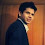# Detecting and Correcting Multicollinearity Problem in Regression Model

Multicollinearity

Multicollinearity means independent variables are highly correlated to each other. In regression analysis, it's an important assumption that regression model should not be faced with a problem of multicollinearity.

Why is multicollinearity a problem?

If the purpose of the study is to see how independent variables impact dependent variable, then multicollinearity is a big problem.

If two explanatory variables are highly correlated, it's hard to tell which has an effect on the dependent variable.
Lets say, Y is regressed against X1 and X2 and where X1 and X2 are highly correlated. Then the effect of X1 on Y is hard to distinguish from the effect of X2 on Y because any increase in X1 tends to be associated with an increase in X2.

Another way to look at multicollinearity problem is : Individual t-test P values can be misleading. It means a P value can be high which means variable is not important, even though the variable is important.

When multicollinearity is not a problem?

1. If your goal is simply to predict Y from a set of X variables, then multicollinearity is not a problem. The predictions will still be accurate, and the overall R2 (or adjusted R2) quantifies how well the model predicts the Y values.
2. Multiple dummy (binary) variables that represent a categorical variable with three or more categories.

How to detect multicollinearity?

Variance Inflation Factor (VIF) - It provides an index that measures how much the variance (the square of the estimate's standard deviation) of an estimated regression coefficient is increased because of collinearity.

VIF = 1 / (1-R-Square of j-th variable) where R2 of jth varible is the coefficient of determination of the model that includes all independent variables except the jth predictor.

Where R-Square of j-th variable is the multiple R2 for the regression of Xj on the other independent variables (a regression that does not involve the dependent variable Y).
If VIF > 5 then there is a problem with multicollinearity.

Interpretation of VIF

If the variance inflation factor of a predictor variable is 5 this means that variance for the coefficient of that predictor variable is 5 times as large as it would be if that predictor variable were uncorrelated with the other predictor variables.

In other words, if the variance inflation factor of a predictor variable is 5 this means that the standard error for the coefficient of that predictor variable is 2.23 times (√5 = 2.23) as large as it would be if that predictor variable were uncorrelated with the other predictor variables.

Correcting Multicollinearity
1. Remove one of highly correlated independent variable from the model.  If you have two or more factors with a high VIF, remove one from the model.
2. Principle Component Analysis (PCA) - It cut the number of interdependent variables to a smaller set of uncorrelated components. Instead of using highly correlated variables, use components in the model that have eigenvalue greater than 1.
3. Run PROC VARCLUS and choose variable that has minimum (1-R2) ratio within a cluster.
4. Ridge Regression - It is a technique for analyzing multiple regression data that suffer from multicollinearity.
5. If you include an interaction term (the product of two independent variables), you can also reduce multicollinearity by "centering" the variables. By "centering", it means subtracting the mean from the independent variables values before creating the products.
For example : Height and Height2 are faced with problem of multicollinearity.
First Step : Center_Height = Height - mean(Height)
Second Step : Center_Height2 = Height2 - mean(Height2)
Third Step : Center_Height3 = Center_Height * Center_Height2
Related Posts
ShareDeepanshu founded ListenData with a simple objective - Make analytics easy to understand and follow. He has over 10 years of experience in data science. During his tenure, he worked with global clients in various domains like Banking, Insurance, Private Equity, Telecom and HR.

5 Responses to "Detecting and Correcting Multicollinearity Problem in Regression Model"
1.How to check multicollinearity for categorical variables?. eg. X1(gender), X2(age group),X3 (income group). Here some correlation will be there in between age group and income level. So how to detect this and how to get rid from this.

1.http://www.listendata.com/2015/04/detecting-multicollinearity-of.html

2.Hi Deppanshu,

How to remove multicollinearity for Categorical variable.

2.How to resolve multicollinearity for interactions? Example: If I have variable X Y Z and XY in the model then I am getting vif>10 for x y and xy.

3.Good to use this explanation.

Next → ← Prev
Looks like you are using an ad blocker!

To continue reading you need to turnoff adblocker and refresh the page. We rely on advertising to help fund our site. Please whitelist us if you enjoy our content.